# Approximation solvability

(diff) ← Older revision | Latest revision (diff) | Newer revision → (diff)

A-solvability

Letand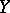be Banach spaces (cf. also Banach space), let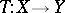be a, possibly non-linear, mapping (cf. also Non-linear operator) and letbe an admissible scheme for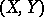, which, for simplicity, is assumed to be a complete projection scheme, i.e.and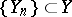are finite-dimensional subspaces with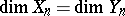for eachandand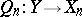are linear projections such thatand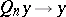forand. Clearly, such schemes exist if bothandhave a Schauder basis (cf. also Basis; Biorthogonal system). Consider the equation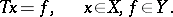(a1)

One of the basic problems in functional analysis is to "solve" (a1). Here, "solvability" of (a1) can be understood in (at least) two manners:

A) solvability in which a solutionof (a1) is somehow established; or

B) approximation solvability of (a1) (with respect to), in which a solutionof (a1) is obtained as the limit (or at least, a limit point) of solutions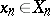of finite-dimensional approximate equations: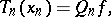(a2)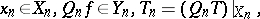with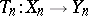continuous for each. If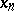andare unique, then (a1) is said to be uniquely A-solvable.

Although the concepts A) and B) are distinct in their purpose, they are not independent. In fact, sometimes knowledge of A) is essential for B) to take place.

Ifandare Hilbert spaces (cf. Hilbert space), the projections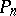and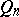are assumed to be orthogonal (cf. Orthogonal projector). If, for example,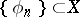andare orthogonal bases, then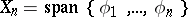and, and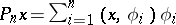and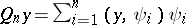for,. In this case, setting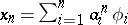, the coefficients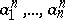are determined by (a2), which reduces to the system## A-proper.

In studying the A-solvability of (a1) one may ask: For what type of linear or non-linear mappingis it possible to show that (a1) is uniquely A-solvable? It turns out that the notion of an A-proper mapping is essential in answering this question.

A mappingis called A-proper if and only ifis continuous for eachand such that ifis any bounded sequence satisfying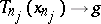for some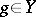, then there exist a subsequenceand ansuch that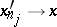as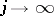and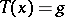, as was first shown in [a2].

It was found (see [a1]) that there are intimate relationships between (unique) A-solvability and A-properness of, shown by the following results:

R1) Ifis a continuous linear mapping, then (a1) is uniquely A-solvable if and only ifis A-proper and one-to-one. This is the best possible result, which includes as a special case all earlier results for the Galerkin or Petrov–Galerkin method (cf. also Galerkin method).

R2) Ifis non-linear and(a3)

for all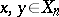,, whereis a continuous function on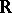with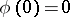,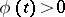for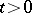and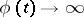as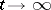, then (a1) is uniquely A-solvable for eachif and only ifis A-proper and one-to-one. Ifis continuous, then R2) holds without the condition thatbe one-to-one. The result R2) includes various results for strongly monotone or strongly accretive mappings (cf. also Accretive mapping). Ifis a continuous linear mapping, then (a3) reduces to(a4)

for all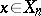,, and some. If, in addition, the scheme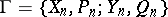is nested, i.e.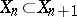andfor all, andin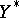for each, thenis A-proper and one-to-one if and only if (a4) holds. In particular, by R1), equation (a1) is uniquely A-solvable for each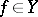. Without this extra condition on, equation (a1) is uniquely A-solvable if (a1) is solvable for each, or if eitheroris reflexive (cf. also Reflexive space).

How to Cite This Entry:
Approximation solvability. Encyclopedia of Mathematics. URL: http://encyclopediaofmath.org/index.php?title=Approximation_solvability&oldid=15238
This article was adapted from an original article by W.V. Petryshyn (originator), which appeared in Encyclopedia of Mathematics - ISBN 1402006098. See original article